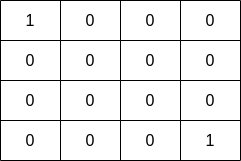2658. Maximum Number of Fish in a Grid
Medium
270
16

You are given a 0-indexed 2D matrix `grid` of size `m x n`, where `(r, c)` represents:

• A land cell if `grid[r][c] = 0`, or
• A water cell containing `grid[r][c]` fish, if `grid[r][c] > 0`.

A fisher can start at any water cell `(r, c)` and can do the following operations any number of times:

• Catch all the fish at cell `(r, c)`, or
• Move to any adjacent water cell.

Return the maximum number of fish the fisher can catch if he chooses his starting cell optimally, or `0` if no water cell exists.

An adjacent cell of the cell `(r, c)`, is one of the cells `(r, c + 1)`, `(r, c - 1)`, `(r + 1, c)` or `(r - 1, c)` if it exists.

Example 1:```Input: grid = [[0,2,1,0],[4,0,0,3],[1,0,0,4],[0,3,2,0]]
Output: 7
Explanation: The fisher can start at cell `(1,3)` and collect 3 fish, then move to cell `(2,3)` and collect 4 fish.
```

Example 2:```Input: grid = [[1,0,0,0],[0,0,0,0],[0,0,0,0],[0,0,0,1]]
Output: 1
Explanation: The fisher can start at cells (0,0) or (3,3) and collect a single fish.
```

Constraints:

• `m == grid.length`
• `n == grid[i].length`
• `1 <= m, n <= 10`
• `0 <= grid[i][j] <= 10`
Accepted
14.4K
Submissions
24.4K
Acceptance Rate
58.9%

Seen this question in a real interview before?
1/4
Yes
No

Discussion (0)

Related Topics